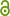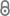# "A variational formulation for fuzzy analysis in continuum mechanics"

R. Mahnken, Mathematics and Mechanics of Complex Systems 5 (2017).https://msp.org/memocs/2017/5-3/memocs-v5-n3-p03-s.pdf
Journal Article | Published | English
Author
Mahnken, Rolf
Department
Abstract
In order to improve the credibility of modern simulation tools, uncertainties of different kinds have to be considered. This work is focused on epistemic uncertainties in the framework of continuum mechanics, which are taken into account by fuzzy analysis. The underlying min-max optimization problem of the extension principle is approximated by α-discretization, resulting in a separation of minimum and maximum problems. To become more universal, so-called quantities of interest are employed, which allow a general formulation for the target problem of interest. In this way, the relation to parameter identification problems based on least-squares functions is highlighted. The solutions of the related optimization problems with simple constraints are obtained with a gradient-based scheme, which is derived from a sensitvity analysis for the target problem by means of a variational formulation. Two numerical examples for the fuzzy analysis of material parameters are concerned with a necking problem at large strain elastoplasticity and a perforated strip at large strain hyperelasticity to demonstrate the versatility of the proposed variational formulation.
Keywords
Publishing Year
Journal Title
Mathematics and Mechanics of complex systems
Volume
5
Issue
3-4
LibreCat-ID

### Cite this

Mahnken R. “A variational formulation for fuzzy analysis in continuum mechanics.” Mathematics and Mechanics of complex systems. 2017;5(3-4).
Mahnken, R. (2017). “A variational formulation for fuzzy analysis in continuum mechanics.” Mathematics and Mechanics of Complex Systems, 5(3–4).
@article{Mahnken_2017, title={“A variational formulation for fuzzy analysis in continuum mechanics”}, volume={5}, number={3–4}, journal={Mathematics and Mechanics of complex systems}, author={Mahnken, Rolf}, year={2017} }
Mahnken, Rolf. “‘A Variational Formulation for Fuzzy Analysis in Continuum Mechanics.’” Mathematics and Mechanics of Complex Systems 5, no. 3–4 (2017).
R. Mahnken, “‘A variational formulation for fuzzy analysis in continuum mechanics,’” Mathematics and Mechanics of complex systems, vol. 5, no. 3–4, 2017.
Mahnken, Rolf. “‘A Variational Formulation for Fuzzy Analysis in Continuum Mechanics.’” Mathematics and Mechanics of Complex Systems, vol. 5, no. 3–4, 2017.
All files available under the following license(s):Closed Access